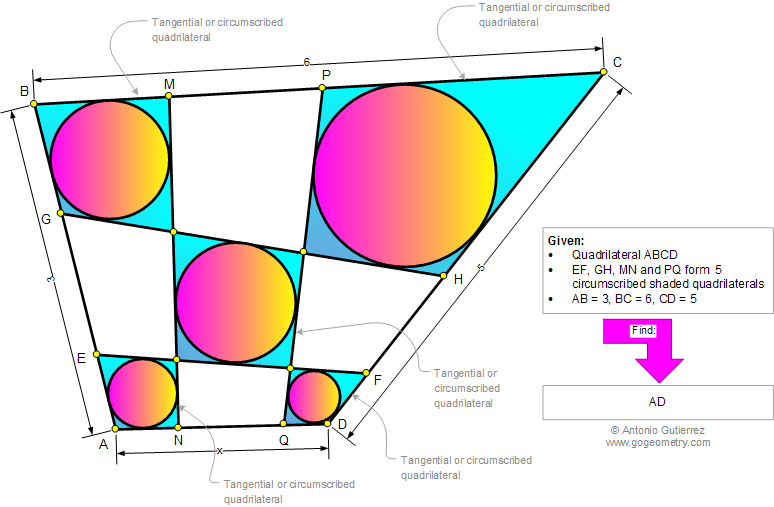# Online Geometry Problem 883: Five Tangential or Circumscribed Quadrilaterals, Circle, Tangent, Common Tangent. Level: High School, Honors Geometry, College, Mathematics Education

 The diagram below shows a quadrilateral ABCD with the points E and G on AB, M and P on BC, H and F on CD, Q and N on AD. Lines EF, GH, MN, and PQ form 5 circumscribed shade quadrilateral, If AB = 3, BC = 6, and CD = 5, find AD.Geometry problem solving is one of the most challenging skills for students to learn. When a problem requires auxiliary construction, the difficulty of the problem increases drastically, perhaps because deciding which construction to make is an ill-structured problem. By “construction,” we mean adding geometric figures (points, lines, planes) to a problem figure that wasn’t mentioned as "given."
 Home | Search | Geometry | Problems | All Problems | Open Problems | Visual Index | 10 Problems | Problems Art Gallery | Art | 881-890 | Circle | Tangential or Circumscribed Quadrilateral | Circle Tangent Line | Solution / comment | By Antonio Gutierrez